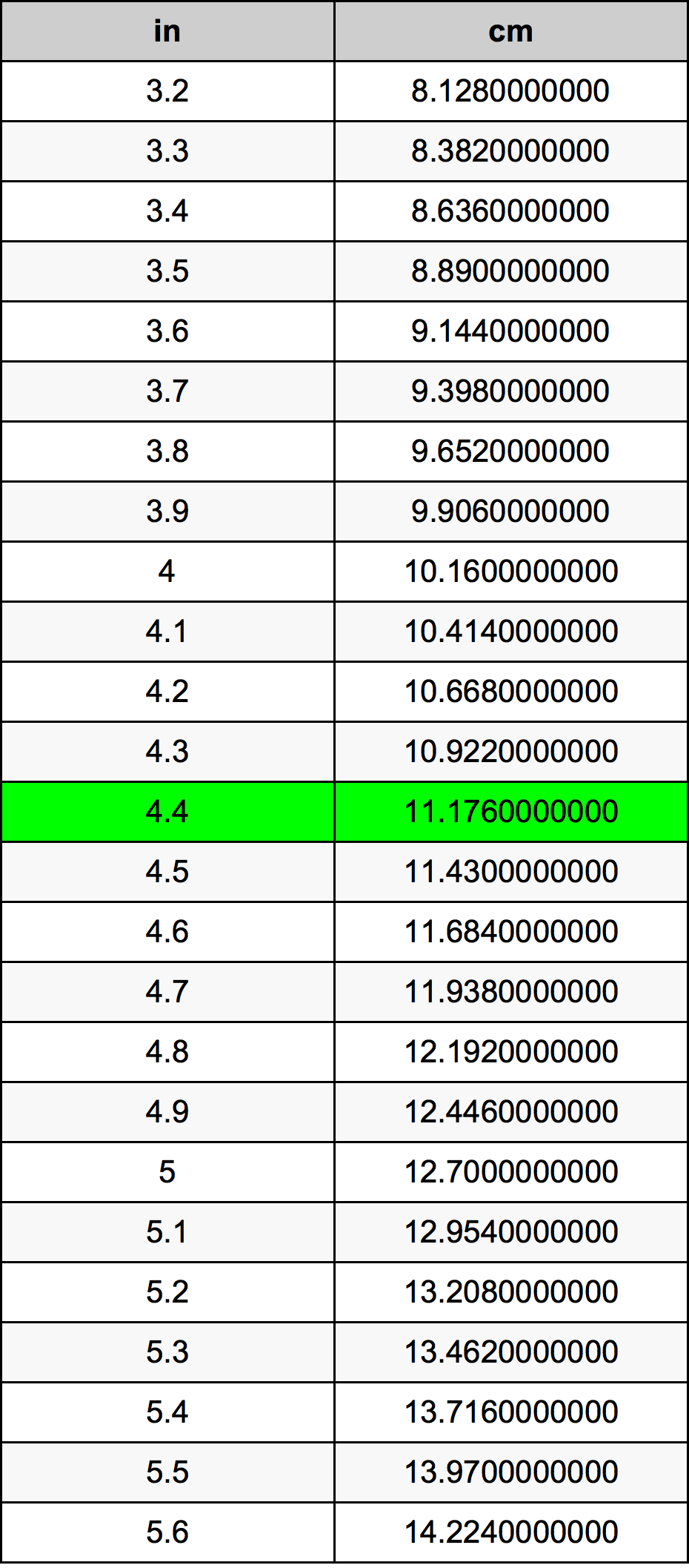Inches To Centimeters

# 4.4 in to cm4.4 Inches to Centimeters

in
=
cm

## How to convert 4.4 inches to centimeters?

 4.4 in * 2.54 cm = 11.176 cm 1 in
A common question is How many inch in 4.4 centimeter? And the answer is 1.7322834646 in in 4.4 cm. Likewise the question how many centimeter in 4.4 inch has the answer of 11.176 cm in 4.4 in.

## How much are 4.4 inches in centimeters?

4.4 inches equal 11.176 centimeters (4.4in = 11.176cm). Converting 4.4 in to cm is easy. Simply use our calculator above, or apply the formula to change the length 4.4 in to cm.

## Convert 4.4 in to common lengths

UnitLengths
Nanometer111760000.0 nm
Micrometer111760.0 µm
Millimeter111.76 mm
Centimeter11.176 cm
Inch4.4 in
Foot0.3666666667 ft
Yard0.1222222222 yd
Meter0.11176 m
Kilometer0.00011176 km
Mile6.94444e-05 mi
Nautical mile6.03456e-05 nmi

## What is 4.4 inches in cm?

To convert 4.4 in to cm multiply the length in inches by 2.54. The 4.4 in in cm formula is [cm] = 4.4 * 2.54. Thus, for 4.4 inches in centimeter we get 11.176 cm.

## 4.4 Inch Conversion Table## Alternative spelling

4.4 in to Centimeter, 4.4 in in Centimeter, 4.4 Inch to Centimeter, 4.4 Inch in Centimeter, 4.4 Inches to Centimeters, 4.4 Inches in Centimeters, 4.4 Inches to Centimeter, 4.4 Inches in Centimeter, 4.4 Inch to cm, 4.4 Inch in cm, 4.4 in to cm, 4.4 in in cm, 4.4 Inches to cm, 4.4 Inches in cm